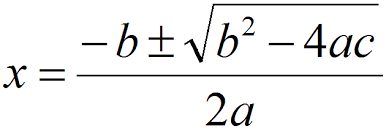`f(x) = (x + 3)(x - 5)`

Then `x = -3` and `x = 5` make `f(x)` zero.The above answer was easy because `f(x)` was factored. The answer is less obvious for

`f(x) = x2 - 2x - 15`

Of course, in general, the coefficients are not integers, which makes the problem even more difficult (unless you have a calculator):

`g(x) = 4.592x2 + 2.308x - 8.083`

Fortunately, the quadratic formula provides a way to find the roots. If the quadratic is

`ax2 + bx + c`

then the formula gives your the roots, if any.

### QUESTION 2:

(Math Review:) Will there be two roots for every choice of `a`, `b`, and `c`?